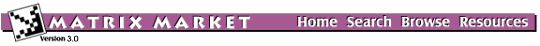## BRUSSEL: Reaction-diffusion Brusselator Model

### from the NEP Collection

Set BRUSSEL
Source: K. Meerbergen, Katholieke Universiteit Leuven, Belgium and A. Spence, University of Bath, UK
Discipline: Chemical engineering

The equations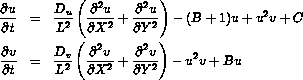for u and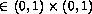with homogeneous Dirichlet boundary conditions form a 2D reaction-diffusion model where u and v represent the concentrations of two reactions. The equations are discretized with central differences with grid size hu = hv =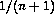with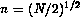. For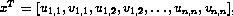, the discretized equations can be written as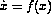. One wants to compute the rightmost eigenvalues of the Jacobi matrix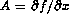, with the parameters B = 5.45, C = 2, Du = 0.004, Dv = 0.008. The parameter L varies.

## Matrices in this set:

The Matrix Market is a service of the Mathematical and Computational Sciences Division / Information Technology Laboratory / National Institute of Standards and Technology.

[ Home ] [ Search ] [ Browse ] [ Resources ]